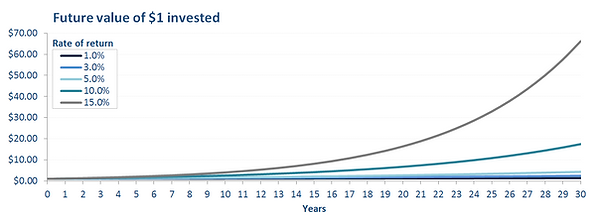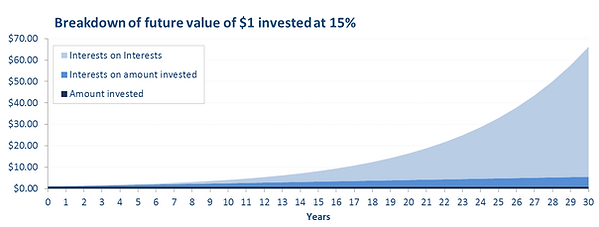top of page

## Finance Basics

Why do we need a discount rate?

Once you have calculated the Free Cash Flows to Firm and/or Free Cash Flows to Equity for the years to come, you need to calculate their present value to assess whether the investment is profitable or how much the company is worth. This can be achieved by discounting the future cash flows. What discounting basically does is that it converts future cash flows into present cash such that it is possible to calculate the value created by a project or the value of an existing asset or company.

Discounting future cash flows allows the investor to take into account:

1. The opportunity cost of undertaking the investment

2. Time value of money (cash available now can be invested at the risk free rate)

3. The risks of a particular project/company relative to the market

4. The financing structure of the asset (especially for cost of equity)

The Weighted Average Cost of Capital (WACC)

There is a very good article on Investopedia that you will find here and that explains how to calculate a WACC.

Discounting cash flows

The choice of the discount rate is key to assess the value of an asset or the potential value creation of a project. Even a small difference in the discount rate can have very large impacts, especially for very long term projects.

To discount a future cash flow, the formula is the following:The graph and the table below show the present value of \$1 received in the future depending on the discount rate used and when this \$1 is received.After only 5 years, \$1 is only worth \$0.50 if discounted at 15% vs \$0.78 if discounted at 5%, it is therefore very important to spend time estimating the cost of capital as it has an equally large impact as the cash flow forecasts.

Excel modeling

1. Calculate the discount factor for each year, the discount factor depends on the base year of the NPV (the year in which you want to calculate the NPV, for this year the discount factor is 1 as it corresponds to the present)2. Multiply each cash flow by the discount factor

3. That's it!The power of compounding interests

Just like for discounting, when investing a given amount, the expected rate of return will have a very large impact on the future amount at a given point in time, this is because of compounding. Compounding is the fact that interests that are earned are reinvested and will subsequently yield additional interests. In the end, these "interests on interests" will account for the largest chunk of the future value and the higher the rate of return, the higher the effect of compounding.bottom of page